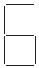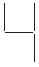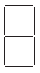# Class 7 Math NCERT Solutions for Chapter – 12 Algebraic Expressions Ex – 12.4

## Algebraic Expressions

1. Observe the patterns of digits made from line segments of equal length. You will find such segmented digits on the display of electronic watches or calculators.

If the number of digits formed is taken to be n, the number of segments required to form n digits is given by the algebraic expression appearing on the right of each pattern. How many segments are required to form 5, 10, 100 digits of the kind

Solution:-

(a) From the question it is given that the numbers of segments required to form n digits of the kindis (5n + 1)

Then,

The number of segments required to form 5 digits = ((5 × 5) + 1)

= (25 + 1)

= 26

The number of segments required to form 10 digits = ((5 × 10) + 1)

= (50 + 1)

= 51

The number of segments required to form 100 digits = ((5 × 100) + 1)

= (500 + 1)

= 501

(b) From the question it is given that the numbers of segments required to form n digits of the kindis (3n + 1)

Then,

The number of segments required to form 5 digits = ((3 × 5) + 1)

= (15 + 1)

= 16

The number of segments required to form 10 digits = ((3 × 10) + 1)

= (30 + 1)

= 31

The number of segments required to form 100 digits = ((3 × 100) + 1)

= (300 + 1)

= 301

(c) From the question it is given that the numbers of segments required to form n digits of the kindis (5n + 2)

Then,

The number of segments required to form 5 digits = ((5 × 5) + 2)

= (25 + 2)

= 27

The number of segments required to form 10 digits = ((5 × 10) + 2)

= (50 + 2)

= 52

The number of segments required to form 100 digits = ((5 × 100) + 1)

= (500 + 2)

= 502

2. Use the given algebraic expression to complete the table of number patterns.

Solution:-

(i) From the table (2n – 1)

Then, 100th term =?

Where n = 100

= (2 × 100) – 1

= 200 – 1

= 199

(ii) From the table (3n + 2)

5th term =?

Where n = 5

= (3 × 5) + 2

= 15 + 2

= 17

Then, 10th term =?

Where n = 10

= (3 × 10) + 2

= 30 + 2

= 32

Then, 100th term =?

Where n = 100

= (3 × 100) + 2

= 300 + 2

= 302

(iii) From the table (4n + 1)

5th term =?

Where n = 5

= (4 × 5) + 1

= 20 + 1

= 21

Then, 10th term =?

Where n = 10

= (4 × 10) + 1

= 40 + 1

= 41

Then, 100th term =?

Where n = 100

= (4 × 100) + 1

= 400 + 1

= 401

(iv) From the table (7n + 20)

5th term =?

Where n = 5

= (7 × 5) + 20

= 35 + 20

= 55

Then, 10th term =?

Where n = 10

= (7 × 10) + 20

= 70 + 20

= 90

Then, 100th term =?

Where n = 100

= (7 × 100) + 20

= 700 + 20

= 720

(v) From the table (n2 + 1)

5th term =?

Where n = 5

= (52) + 1

= 25+ 1

= 26

Then, 10th term =?

Where n = 10

= (102) + 1

= 100 + 1

= 101

So the table is completed below.

#### Class 7 Math NCERT Solutions for Chapter – 13 Exponents and Powers Ex – 13.1

Lorem ipsum dolor sit amet, consectetur adipiscing elit. Phasellus cursus rutrum est nec suscipit. Ut et ultrices nisi. Vivamus id nisl ligula. Nulla sed iaculis ipsum.

Company Name